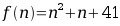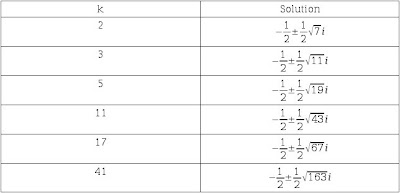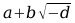## Euler's Prime Generating Equation

In the eighteenth century, Leonhard Euler discovered (no one knows how) that the equationproduced primes consecutively when fed with numbers n=0 to 39. Even though the equation fails when n=40 it still manages to spit out more prime numbers than any other quadratic equation. Of the first 10 million values the proportion of primes is about one in three. Euler then showed that you can substitute 41 with k=2,3,5,11,17 and the formulawould still produce primes from n=0 to k-2. To show you why this equation is so special I'm going to first solve the quadratic equation for all the values of k.The numbers 7, 11, 19, 43, 67, 163 are what make the equation special, but what's so specials about these numbers you ask?

Gaussian Integers and the Class Number Problem
In 1796 Carl Friedrich Gauss proved a theorem called the quadratic reciprocity law, which concerns the solutions to equations of the formwhere p and q are prime numbers. Gauss found that his calculations were made easier if he worked with numbers of the form a+bi, where a and b are integers. These numbers are now known as Gaussian integers. These numbers turned out to be very useful in other areas of maths. Gauss then investigated other systems with which you could create a 'number theory' out of, one of the systems he looked at was of the formwhere d is some integer other than 1. Here is a list of the values of d which produce a unique factorisation theorem.

d=1, 2, 3, 7, 11, 19, 43, 67, 163

Notice anything familiar? In 1952, Kurt Heegner managed to prove that d=163 was the biggest value that could make it work. And so there is the reason why those numbers and that equation are so special.